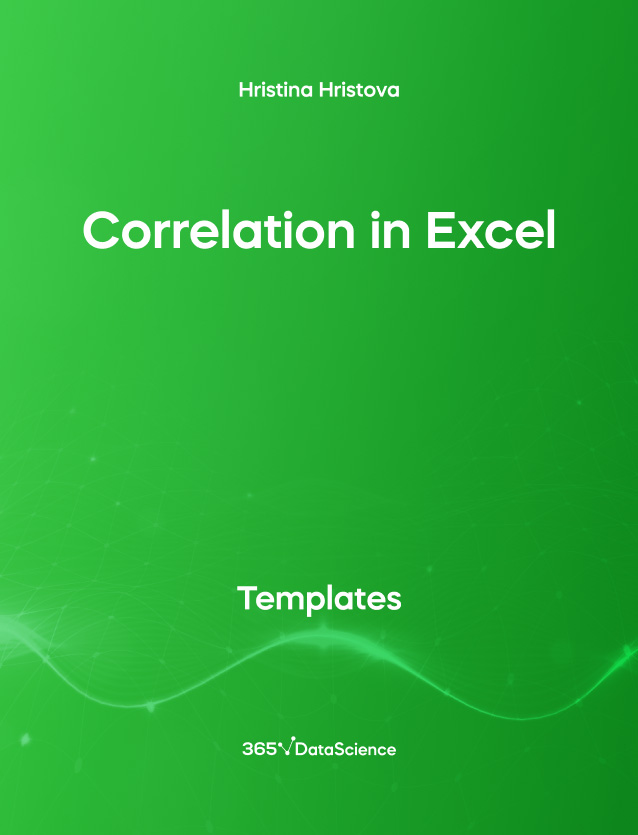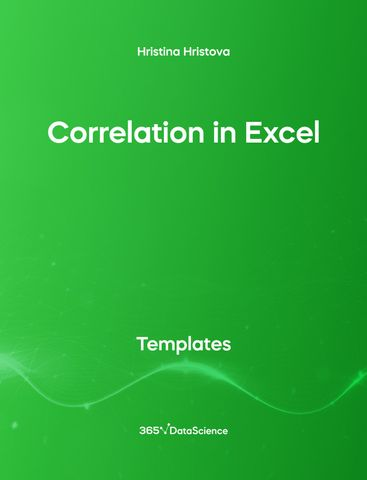# Correlation in Excel TemplateHristina Hristova

Course Author
The Correlation in Excel template demonstrates how the correlation coefficient can be calculated in Excel. Some other related topics you might be interested in are Calculating the Variance in Excel, Standard Deviation in Excel, Coefficient of Variation in Excel, Covariance in Excel. You can now download the Excel template for free. The Correlation in Excel template is among the topics covered in detail in the 365 Data Science program.

## Who is it for

This is an open-access Excel template that is going to be very helpful for Data Scientists, Data Analysts, Business Analysts and anyone who wants to know how to calculate the correlation in Excel.